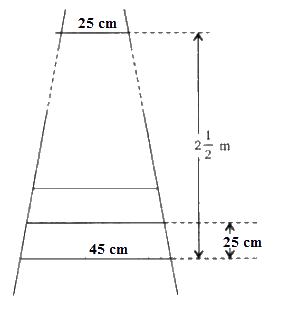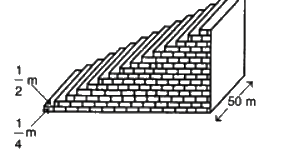# NCERT Solutions For Class 10 Maths Chapter 5 Exercise 5.4

Go back to  'Arithmetic Progressions'

## Chapter 5 Ex.5.4 Question 1

Which term of the A.P. $$121, 117, 113 ...$$is its first negative term?

[Hint: Find $$n$$ for $${a_n} < 0$$]

### Solution

What is Known?

A.P. Series: $$121,\, 117, \,113 ...$$

What is Unknown?

First negative term of the AP

Reasoning:

$$n\rm{th}$$ term of an AP is $$\,{a_n} = a + \left( {n - 1} \right)d.$$

Where $$a$$ is the first term, $$d$$ is the common difference and $$n$$ is the number of terms.

Steps:

Given:

• First Term, $$a = 121$$
• Common difference, $$d = 117 - 121 = - 4$$

We know that $$n^\rm{th}$$ term of AP, $${a_n} = a + \left( {n - 1} \right)d$$

\begin{align}{a_n} &= 121 + \left( {n - 1} \right) \times \left( { - 4} \right)\\ &= 121 - 4n + 4\\ &= 125 - 4n\end{align}

For the first negative term of this A.P,

\begin{align}{a_n} &< 0\\125 - 4n &< 0\\125 &< 4n\\n &> \frac{{125}}{4}\\n &> 31.25\end{align}

Therefore, $$32\rm{nd}$$ term will be the first negative term of this A.P.

## Chapter 5 Ex.5.4 Question 2

The sum of the third and the seventh terms of an A.P is $$6$$ and their product is $$8.$$ Find the sum of first sixteen terms of the A.P.

### Solution

What is Known?

$${a_3} + {a_7} = 6$$ and $${a_3} \times {a_7} = 8$$

What is Unknown?

Sum of first $$16$$ terms, $${S_{16}}$$

Reasoning:

Sum of the first $$n$$ terms of an AP is given by $${S_n} = \frac{n}{2}\left[ {2a + \left( {n - 1} \right)d} \right]$$ and $$nth$$ term of an AP is $$\,{a_n} = a + \left( {n - 1} \right)d$$

Where $$a$$ is the first term, $$d$$ is the common difference and $$n$$ is the number of terms.

Steps:

Given:

$${a_3} + {a_7} = 6 \qquad \dots {\rm{ Equation}}\left( 1 \right)$$

$${a_3} \times {a_7} = 8 \qquad\dots {\rm{Equation }}\left( 2 \right)$$

We know that $$n^\rm{th}$$ term of AP Series,

$$\,{a_n} = a + \left( {n - 1} \right)d$$

Third term, $$\,{a_3} = a + \left( {3 - 1} \right)d$$

$$\,{a_3} = a + 2d \qquad\dots\left( 3 \right)$$

Seventh term, $$\,{a_7} = a + \left( {7 - 1} \right)d$$

$$\,{a_7} = a + 6d \quad \dots {\rm{ Equation}}\left( 4 \right)$$

Using Equation (3) and Equation (4) in Equation (1)

\begin{align}\left[ \begin{array} & \left( a+2d \right)+ \\ \left( a+6d \right) \\ \end{array} \right]&=6 \\ 2a+8d&=6 \\ a+4d&=3 \\ a &=3-4d\,\,\,\,\ldots\left( \text{5} \right) \\ \end{align}

Using Equation (3) and Equation (4) in Equation (2)

$\left( {a + 2d} \right) \times \left( {a + 6d} \right) = 8$

Substituting the value of Equation (5) in above,

\begin{align} \left[ \begin{array} & \left( 3-4d+2d \right)\times \\ \left( 3-4d+6d \right) \\ \end{array} \right]&=8 \\ \left[ \begin{array} & \left( 3-2d \right)\times \\ \left( 3+2d \right) \\ \end{array} \right]&=8 \\ {{\left( 3 \right)}^{2}}-{{\left( 2d \right)}^{2}}&=8 \\ 9-4{{d}^{2}}&=8 \\ 4{{d}^{2}}&=1 \\ {{d}^{2}}&=\frac{1}{4} \\d&=\pm \frac{1}{2} \\d&=\frac{1}{2}\ \text{or}\ \left( -\frac{1}{2} \right) \\ \end{align}

Hence by substituting both the values of $$d,$$

When $$d = \frac{1}{2}$$

\begin{align}a &= 3 - 4d\\ &= 3 - 4 \times \frac{1}{2}\\& = 3 - 2\\ &= 1\end{align}

When $$d = - \frac{1}{2}$$

\begin{align}a &= 3 - 4d\\ &= 3 - 4 \times \left( { - \frac{1}{2}} \right)\\ &= 3 + 2\\& = 5\end{align}

We know that Sum of $$n^\rm{th}$$ term of AP Series, $${S_n} = \frac{n}{2}\left[ {2a + \left( {n - 1} \right)d} \right]$$

When $$a = 1$$ and $$d = \frac{1}{2}$$

\begin{align}{S_{16}} &= \frac{{16}}{2}\left[ {2 \times 1 + \left( {16 - 1} \right) \times \frac{1}{2}} \right]\\ &= 8\left[ {2 + \frac{{15}}{2}} \right]\\ &= 8 \times \frac{{19}}{2}\\ &= 76\end{align}

When $$a = 5$$ and $$d = - \frac{1}{2}$$

\begin{align}{S_{16}} &\!=\!\frac{{16}}{2}\left[ {2\!\times\! 5\!+\!\left( {16\!-\!1} \right) \!\times\!\! \left( { - \frac{1}{2}} \right)} \right]\\ &= 8\left[ {10 - \frac{{15}}{2}} \right]\\ &= 8 \times \frac{5}{2}\\&= 20\end{align}

Therefore, $${S_{16}} = 20,76$$

## Chapter 5 Ex.5.4 Question 3

A ladder has rungs $$25\,\rm{ cm}$$ apart. (See figure). The rungs decrease uniformly in length from $$45\,\rm{ cm}$$ at the bottom to $$25\,\rm{ cm}$$ at the top. If the top and bottom rungs are \begin{align} 2\frac{1}{2} \,\rm{m} \end{align} apart, what is the length of the wood required for the rungs?

[Hint: number of rungs = $$\frac{{250}}{{25}}$$ ]### Solution

What is Known?

The rungs decrease uniformly in length from $$45\,\rm{ cm}$$ at the bottom to $$25\,\rm{ cm}$$ at the top and the top and bottom rungs are $$2\frac{1}{2}\,\rm{m}$$ apart.

What is Unknown?

The length of the wood required for the rungs

Reasoning:

Sum of the first $$n$$ terms of an AP is given by $${S_n} = \frac{n}{2}\left[ {a + l} \right]$$

Where $$a$$ is the first term, $$l$$ is the last term and $$n$$ is the number of terms.

Steps:

Given:

• Distance between the rungs $$= 25\,\rm{cm}$$
• Distance between the top and bottom rungs \begin{align}=2\frac{1}{2} \;\rm{m} = 2\frac{1}{2} \times100 \,\rm{cm}\end{align}

$$\therefore$$ Total number of rungs

\begin{align}&= \frac{{2\frac{1}{2} \times 100}}{{25}} + 1\\&= \frac{{250}}{{25}} + 1 \\&= 11\end{align}

From the given Figure, we can observe that the lengths of the rungs decrease uniformly, hence we can conclude that they will be in an AP

The length of the wood required for the rungs equals the sum of all the terms of this A.P.

• First term, $$a = 45$$
• Last term, $$l = 25$$
• Number of terms, $$n = 11$$

Hence Sum of $$n$$ terms of the AP Series,

\begin{align}{S_n} &= \frac{n}{2}\left[ {a + l} \right]\\{S_{11}} &= \frac{{11}}{2}\left[ {45 + 25} \right]\\ &= \frac{{11}}{2} \times 70\\&= 11 \times 35\\&= 385\end{align}

Therefore, the length of the wood required for the rungs is $$385 \,\rm{cm.}$$

## Chapter 5 Ex.5.4 Question 4

The houses of a row are number consecutively from $$1$$ to $$49.$$ Show that there is a value of $$x$$ such that the sum of numbers of the houses preceding the house numbered $$x$$ is equal to the sum of the number of houses following it. Find this value of $$x.$$

[Hint: $${S_x}_{ - 1} = {S_{49}} - {S_x}$$]

### Solution

What is Known?

The houses of a row are number consecutively from $$1$$ to $$49$$

What is Unknown?

Value of $$x$$

Reasoning:

Sum of the first $$n$$ terms of an AP is given by $${S_n} = \frac{n}{2}\left[ {2a + \left( {n - 1} \right)d} \right]$$

Where $$a$$ is the first term, $$d$$ is the common difference and $$n$$ is the number of terms.

Steps:

Given:

Number of houses are $$1,2,3, .................49$$

By Observation, the numbers of houses are in an A.P.

Hence

• First term, $$a = 1$$
• Common difference, $$d = 1$$

Let us assume that the number of $$x^\rm{th}$$ house can be expressed as below:

We know that ,

Sum of n terms in an A.P. $${S_n} = \frac{n}{2}\left[ {2a + \left( {n - 1} \right)d} \right]$$

Sum of numbers of houses preceding $$x^\rm{th}$$ house $$= S_{x−1}$$

\begin{align}{S_{x - 1}} &\!=\!\frac{{\left( {x - 1} \right)}}{2}\left[\!{2a \!+\!\left( {\left( {x\!-\!1} \right) \!- 1} \right)d} \right]\\& = \frac{{\left( {x\!-\!1} \right)}}{2}\left[ {2\!\times\! 1\!+\!\left( {x\!-\!2} \right) \!\times\! 1} \right]\\ &= \frac{{\left( {x - 1} \right)}}{2}\left[ {2 + x - 2} \right]\\ &= \frac{{x\left( {x - 1} \right)}}{2}\end{align}

By the given we know that, Sum of number of houses following $$x^\rm{th}$$ house $$= S_{49} – S_{x}$$

\begin{align} {{S}_{49}}-{{S}_{x}}&=\left\{ \begin{array} & \frac{49}{2}\left[ 2\times 1+\left( 49-1 \right)\times 1 \right] \\ - \frac{x}{2}\left[ 2\times 1+\left( x-1 \right)\times 1 \right] \\ \end{array} \right\} \\ & =\frac{49}{2}\left[ 2+48 \right]-\frac{x}{2}\left[ 2+x-1 \right] \\ & =\frac{49}{2}\left[ 2+48 \right]-\frac{x}{2}\left[ 2+x-1 \right] \\ & =\frac{49}{2}\times 50-\frac{x}{2}\left[ x+1 \right] \\ & =1225-\frac{x\left( x+1 \right)}{2} \\ \end{align}

It is given that these sums are equal.

\begin{align}\frac{{x\left( {x - 1} \right)}}{2} &= 1225 - \frac{{x\left( {x + 1} \right)}}{2}\\\frac{{{x^2}}}{2} - \frac{x}{2} &= 1225 - \frac{{{x^2}}}{2} - \frac{x}{2}\\{x^2} &= 1225\\x &= \pm 35\end{align}

As the number of houses cannot be a negative number, we consider number of houses, $$x = 35$$

Therefore, house number $$35$$ is such that the sum of the numbers of houses preceding the house numbered $$35$$ is equal to the sum of the numbers of the houses following it.

## Chapter 5 Ex.5.4 Question 5

A small terrace at a football ground comprises of $$15$$ steps each of which is $$50\, \rm{m}$$ long and built of solid concrete. Each step has a rise of \begin{align}\frac{1}{4}\,\rm{m} \end{align} and a tread of \begin{align}\frac{1}{2}\,\rm{m} \end{align} (See figure) calculate the total volume of concrete required to build the terrace.### Solution

What is Known?

$$15$$ steps each of which is $$50 \,\rm{m}$$ long and each step has a rise of \begin{align} \frac{1}{4}\,\rm{m} \end{align} and a tread of \begin{align} \frac{1}{2}\,\rm{m} \end{align}

What is Unknown?

Total volume of concrete required to build the terrace.

Reasoning:

Sum of the first $$n$$ terms of an AP is given by \begin{align} {S_n} = \frac{n}{2}\left[ {2a + \left( {n - 1} \right)d} \right] \end{align}

Where $$a$$ is the first term, $$d$$ is the common difference and $$n$$ is the number of terms.

Steps:

From the figure, it can be observed that

Height of $$1^{st}$$ step is \begin{align}\frac{1}{4} \,\rm{m} \end{align}

Height of $$2^{nd}$$ step is \begin{align} \left( {\frac{1}{4} + \frac{1}{4}} \right) \,\rm{m} = \frac{1}{2} \,\rm{m} \end{align}

Height of $$3^{rd}$$ step is \begin{align} \left( {\frac{1}{2} + \frac{1}{4}} \right)\,\rm{m} = \frac{3}{4} \,\rm{m} \end{align}

Therefore, height of the each step is increasing by \begin{align} \frac{1}{4} \,\rm{m} \end{align}  length $$50 \,\rm{m}$$ and width (tread) \begin{align} \frac{1}{2} \,\rm{m} \end{align} remain the same for each of the steps.

Volume of Step can be considered as Volume of Cuboid= Length $$\times$$ Breadth $$\times$$ Height

Volume of concrete in $$1^{st}$$ step \begin{align}\!=\!50m \!\times\! \frac{1}{2} \,\rm{m} \!\times\! \frac{1}{4} \,\rm{m} \!=\! 6.25\,{m^3} \end{align}

Volume of concrete in $$2^{nd}$$ step \begin{align} = 50 \,\rm{m} \times \frac{1}{2} \,\rm{m} \times \frac{1}{2} \,\rm{m} = 12.50\,{m^3} \end{align}

Volume of concrete in $$3^{rd}$$ step \begin{align} = 50 \,\rm{m} \times \frac{1}{2} \,\rm{m} \times \frac{3}{4} \,\rm{m} = 18.75\,{m^3} \end{align}

It can be observed that the volumes of concrete in these steps are in an A.P.

$6.25\,\rm{m^3},12.50\,\rm{m^3},\,18.75\,\rm{m^3},.......$

• First term, $$a = 6.25$$
• Common difference, $$d = 6.25$$
• Number of steps, $$n = 15$$

Sum of n terms, \begin{align} {S_n} = \frac{n}{2}\left[ {2a + \left( {n - 1} \right)d} \right] \end{align}

\begin{align}{S_{15}} &\!\!=\!\!\frac{{15}}{2}\left[ {2\!\times\!6.25\!+\!\left( {15\!-\!\!1} \right)\!\times\!6.25} \right]\\&= \frac{{15}}{2}\left[ {12.50 + 14 \times 6.25} \right]\\ &= \frac{{15}}{2}\left[ {12.50 + 87.50} \right]\\ &= \frac{{15}}{2} \times 100\\& = 750\end{align}

Therefore, Volume of concrete required to build the terrace is $$750\;\rm{m^3}$$.